The purpose of this lesson is to explain the concept of probability density and likelihood, using a simple example of Normal probability density function.

For those with R studio, here are some optional code and libraries for interactive graphics:

library(manipulate)

source("https://raw.githubusercontent.com/petrkeil/ML_and_Bayes_2017_iDiv/master/Univariate%20Normal%20Model/plotting_functions.r")

# 1 The data

We will use data on housefly wing length [mm], which you can find here.

The original data are from Sokal & Hunter (1955) A morphometric analysis of DDT-resistant and non-resistant housefly strains Ann. Entomol. Soc. Amer. 48: 499-507.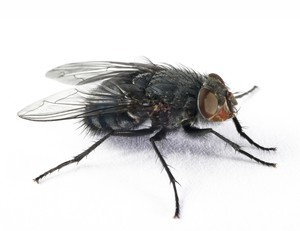housefly

To fetch the data directly from the web, run:

wings <- read.table("http://goo.gl/4lPBG6", header=FALSE)[,1]*0.1

Let’s examine the data:

wings
##    3.6 3.7 3.8 3.8 3.9 3.9 4.0 4.0 4.0 4.0 4.1 4.1 4.1 4.1 4.1 4.1 4.2
##   4.2 4.2 4.2 4.2 4.2 4.2 4.3 4.3 4.3 4.3 4.3 4.3 4.3 4.3 4.4 4.4 4.4
##   4.4 4.4 4.4 4.4 4.4 4.4 4.5 4.5 4.5 4.5 4.5 4.5 4.5 4.5 4.5 4.5 4.6
##   4.6 4.6 4.6 4.6 4.6 4.6 4.6 4.6 4.6 4.7 4.7 4.7 4.7 4.7 4.7 4.7 4.7
##   4.7 4.8 4.8 4.8 4.8 4.8 4.8 4.8 4.8 4.9 4.9 4.9 4.9 4.9 4.9 4.9 5.0
##   5.0 5.0 5.0 5.0 5.0 5.1 5.1 5.1 5.1 5.2 5.2 5.3 5.3 5.4 5.5
par(mfrow=c(1,2))
hist(wings, freq=TRUE, col="grey", main="Frequency histogram")
points(wings, jitter(rep(0, times=100), factor=10))
hist(wings, freq=FALSE, col="grey", main="Density histogram")
points(wings, jitter(rep(0, times=100), factor=0.7))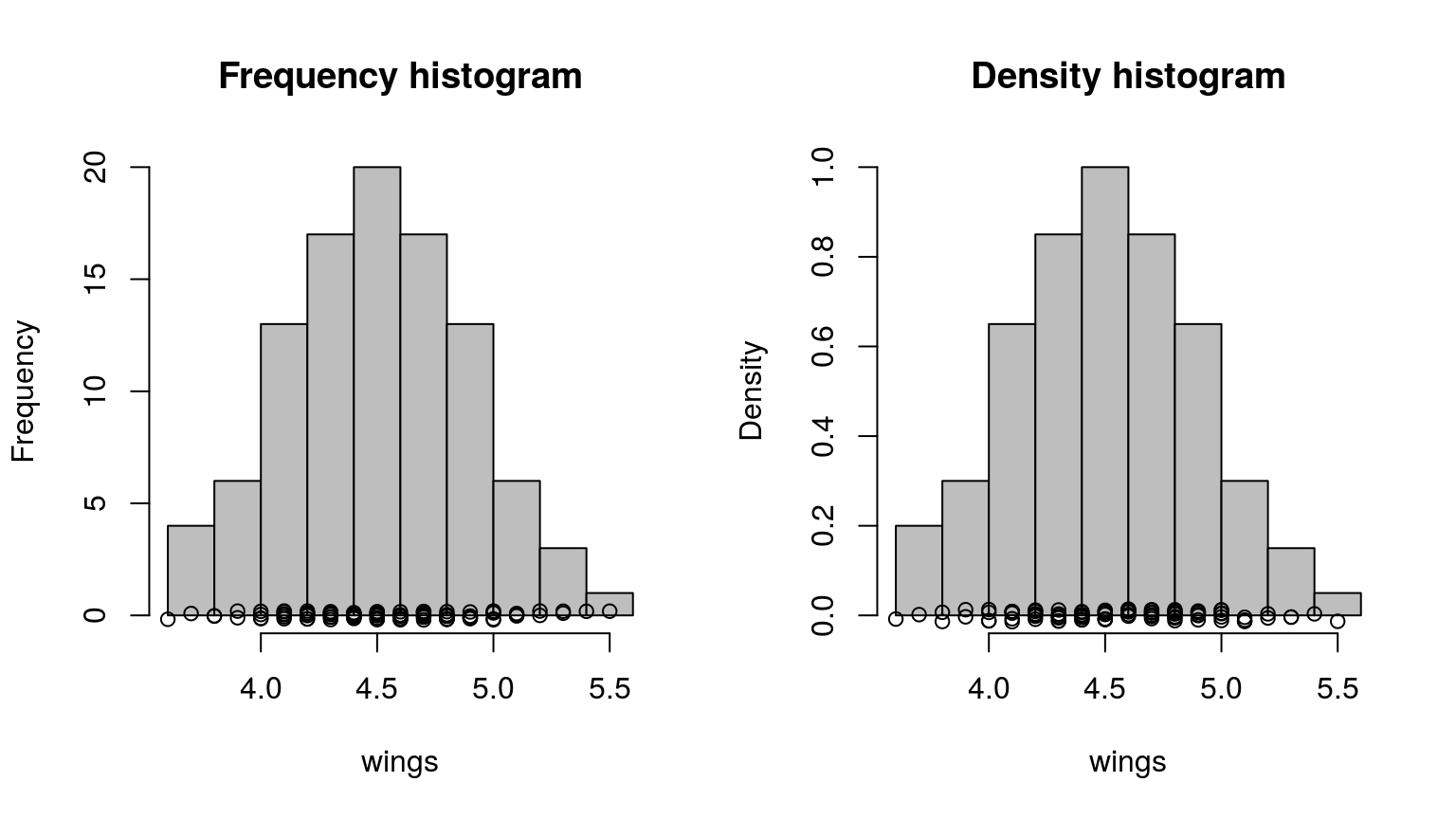# 2 The Normal model

This is our formal model definition:

$p(x) = \frac{1}{\sqrt{2\pi}\sigma}e^{-(x-\mu)^2/(2\sigma^2)}$

where $$p()$$ is probability density function (also known as PDF). The function has two parameters: $$\mu$$ (mean) and $$\sigma$$ (standard deviation).

For $$\mu = 0$$ and $$\sigma = 1$$ the model looks like this:

  curve(dnorm, from=-4, to=4, ylab="p(x)")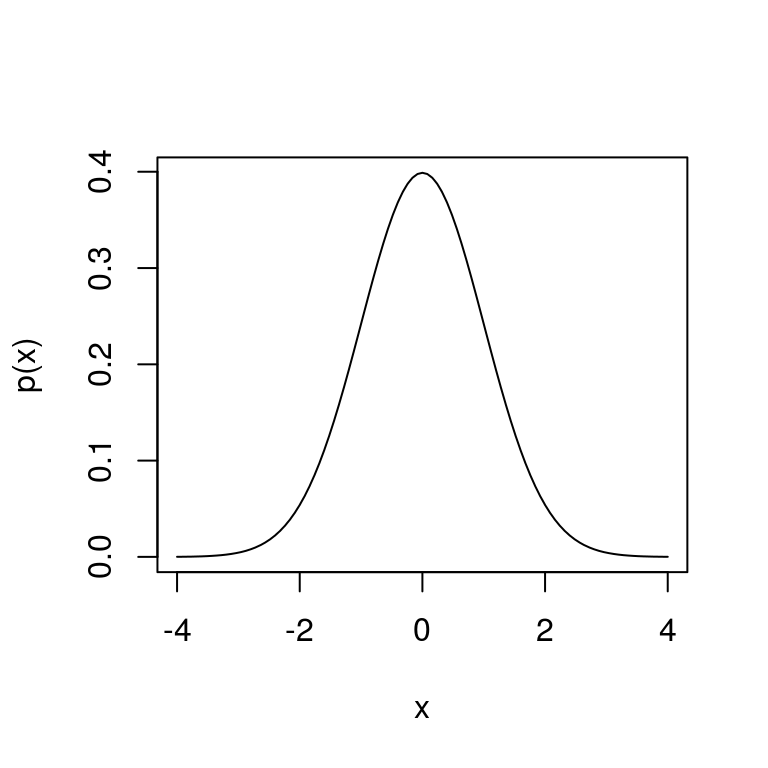Remember:

• Probability density is different from probability.

• Probability density is denoted by $$p$$, while probability is $$P$$.

• Probability density can be higher than 1.

• Probability density function must integrate to 1.

• Probability distribution is an ambiguous term.

# 3 Fitting the model to the wing data

KEY PROBLEM: How do we decide which parametrization is the best for our data?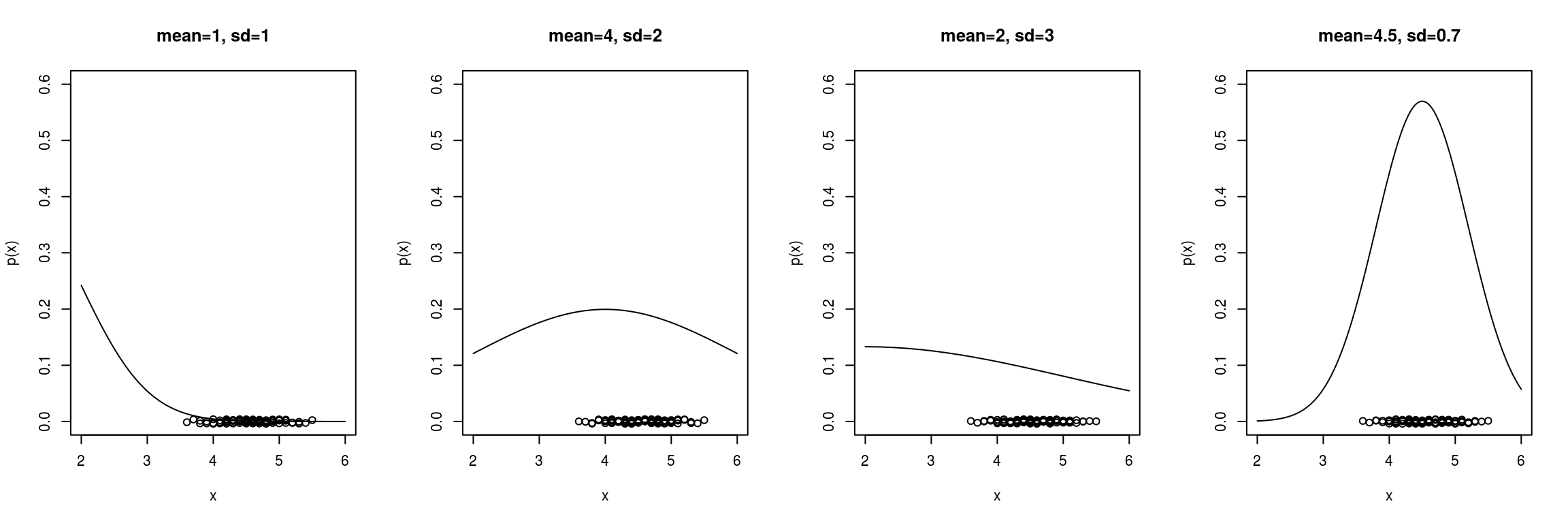We can make this interactive using manipulate:

manipulate(curve.data(mean, sd),
mean=slider(2, 7, step=0.01),
sd=slider(0.1,3, step=0.01, initial=1) )

## 3.1 Likelihood - single data point

The likelihood function is the density evaluated at the data $$x_1$$, … ,$$x_n$$, viewed as a function of model parameters ($$\mu$$ and $$\sigma$$ in case of the Normal model). We write it as $$L(\mu, \sigma | x) = p(x | \mu, \sigma)$$.

Calculation of likelihood in R is easy! The R functions dnorm(), dpois(), dunif(), dbinom(), dgamma(), dbeta() etc. are exactly for that!

Example: What is the likelihood of the first data value in the wings dataset, given the $$Normal(\mu=4, \sigma=1)$$ model?

my.mean = 4
my.sd = 1

Here is the data point that we will examine. Note that I use the letter i to denote index:

wings.i <- wings
wings.i
##  3.6

Here is how you calculate the likelihood for the data point wings.i using the function dnorm:

L <- dnorm(x=wings.i, mean=my.mean, sd=my.sd)
L
##  0.3682701

Let’s plot it: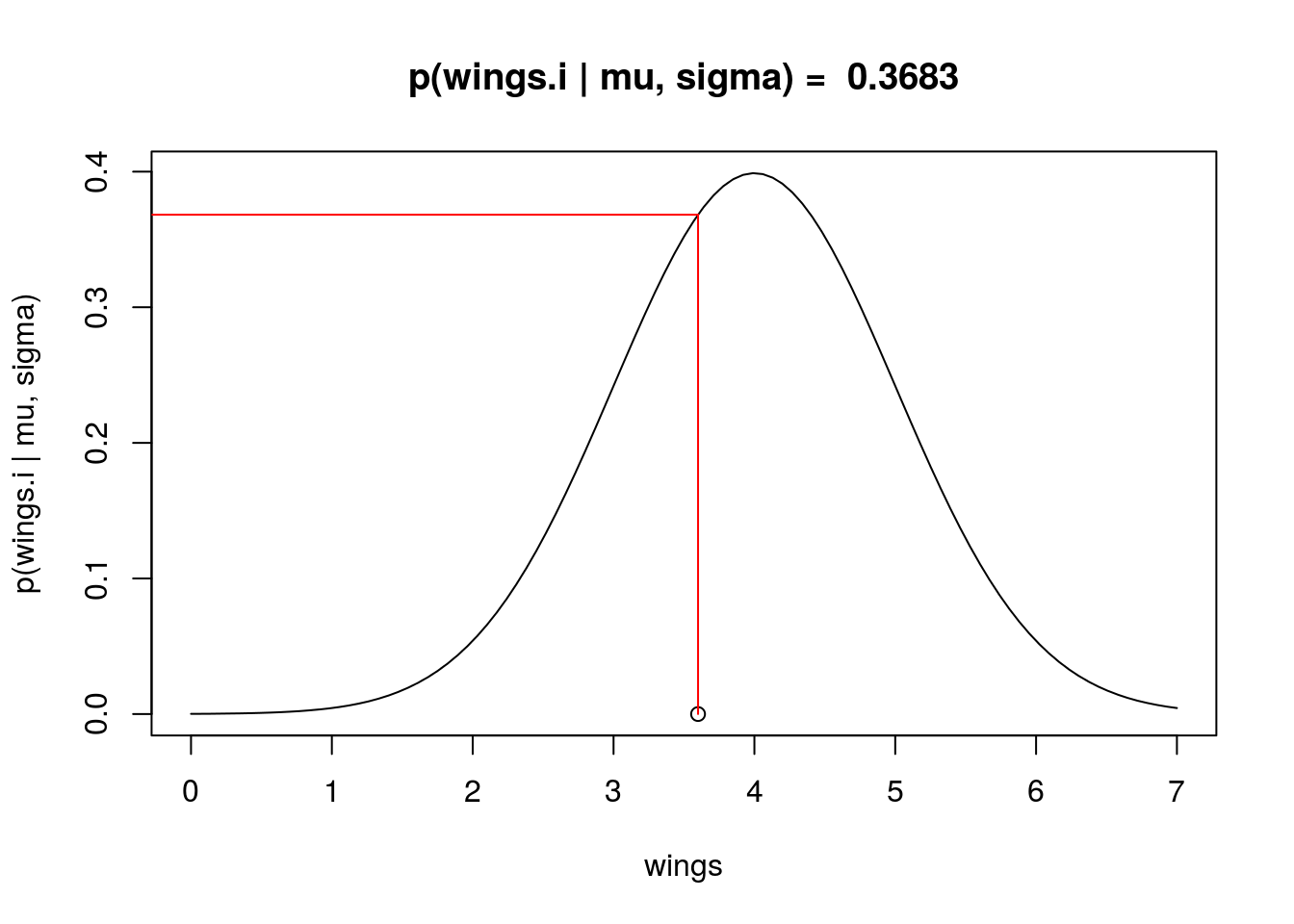You can try some interactive graphics:

manipulate(curve.data.l.point(mean, sd, point),
point = slider(1, length(wings)),
mean=slider(2, 7, step=0.01),
sd=slider(0.1,3, step=0.01, initial=1) )

# 4 Likelihood - whole dataset

Basic probability theory tells us that:

$P(A \cap B) = P(A) \times P(B|A) = P(B) \times P(A|B)$

Where $$P(A \cap B)$$ is joint probability, associated with AND, meaning “at the same time”.

The problem is that joint probability for more than two events, e.g. $$P( A \cap B \cap C \cap D )$$, can be almost impossible to calculate, with the exception of A and B being independent! Then: $P(A \cap B) = P(A) \times P(B)$ and hence $P( A \cap B \cap C \cap D ) = P(A) \times P(B) \times P(C) \times P(D)$

It follows that it is useful to subject probability density $$p()$$ to the same rules as probability $$P()$$. Hence, we can calculate the likelihood of the whole dataset as a product of likelihoods of all individual data points!

  wings
##    3.6 3.7 3.8 3.8 3.9 3.9 4.0 4.0 4.0 4.0 4.1 4.1 4.1 4.1 4.1 4.1 4.2
##   4.2 4.2 4.2 4.2 4.2 4.2 4.3 4.3 4.3 4.3 4.3 4.3 4.3 4.3 4.4 4.4 4.4
##   4.4 4.4 4.4 4.4 4.4 4.4 4.5 4.5 4.5 4.5 4.5 4.5 4.5 4.5 4.5 4.5 4.6
##   4.6 4.6 4.6 4.6 4.6 4.6 4.6 4.6 4.6 4.7 4.7 4.7 4.7 4.7 4.7 4.7 4.7
##   4.7 4.8 4.8 4.8 4.8 4.8 4.8 4.8 4.8 4.9 4.9 4.9 4.9 4.9 4.9 4.9 5.0
##   5.0 5.0 5.0 5.0 5.0 5.1 5.1 5.1 5.1 5.2 5.2 5.3 5.3 5.4 5.5
  L <- dnorm(x=wings, mean=my.mean, sd=my.sd)
L
##    0.3682701 0.3813878 0.3910427 0.3910427 0.3969525 0.3969525 0.3989423
##    0.3989423 0.3989423 0.3989423 0.3969525 0.3969525 0.3969525 0.3969525
##   0.3969525 0.3969525 0.3910427 0.3910427 0.3910427 0.3910427 0.3910427
##   0.3910427 0.3910427 0.3813878 0.3813878 0.3813878 0.3813878 0.3813878
##   0.3813878 0.3813878 0.3813878 0.3682701 0.3682701 0.3682701 0.3682701
##   0.3682701 0.3682701 0.3682701 0.3682701 0.3682701 0.3520653 0.3520653
##   0.3520653 0.3520653 0.3520653 0.3520653 0.3520653 0.3520653 0.3520653
##   0.3520653 0.3332246 0.3332246 0.3332246 0.3332246 0.3332246 0.3332246
##   0.3332246 0.3332246 0.3332246 0.3332246 0.3122539 0.3122539 0.3122539
##   0.3122539 0.3122539 0.3122539 0.3122539 0.3122539 0.3122539 0.2896916
##   0.2896916 0.2896916 0.2896916 0.2896916 0.2896916 0.2896916 0.2896916
##   0.2660852 0.2660852 0.2660852 0.2660852 0.2660852 0.2660852 0.2660852
##   0.2419707 0.2419707 0.2419707 0.2419707 0.2419707 0.2419707 0.2178522
##   0.2178522 0.2178522 0.2178522 0.1941861 0.1941861 0.1713686 0.1713686
##   0.1497275 0.1295176
  prod(L)
##  1.657651e-50

This is a ridiculously small number! Which is why we have the Negative Log Likelihood:

  - sum(log(L))
##  114.6239

We can encapsulate it into a single function:

  neg.LL.function <- function(x, mean, sd)
{
LL <- dnorm(x=x, mean=mean, sd=sd, log=TRUE) # note the log!!!
neg.LL <- - sum(LL)
return(neg.LL)
}

It’s a function of model parameters, so try to play around with different paramter values:

  neg.LL.function(wings, mean=0, sd=1)
##  1134.624

Negative log-likelihood can be then minimized (likelihood is maximized) in order to find the most likely model parameters - these are the Maximum Likelihood Estimators (MLE) of model parameters.

# 5 Exercise

Find and plot the MLE of the Normal model and the ‘wings’ data.

Use the following functions and scripts:

 neg.LL.function()
hist()
curve()

You can try some interactive graphics:

manipulate(curve.data.LL(mean, sd),
mean=slider(2, 7, step=0.01),
sd=slider(0.1,3, step=0.01, initial=1) )

# 6 Using optim() to find MLE

optim needs the neg.LL.function to take only one object, which can be a vector (or a list). So I will just put all of the data into dat object, and the parameters into par object.

neg.LL.function.for.optim <- function(par, dat)
{
LL <- dnorm(x=dat, mean=par, sd=par, log=TRUE) # note the log!!!
neg.LL <- - sum(LL)
return(neg.LL)
}

And run the actual optimization:

optim(par=c(mean=0,var=1),
fn=neg.LL.function.for.optim,
dat=wings)
## Warning in dnorm(x = dat, mean = par, sd = par, log = TRUE): NaNs
## produced

## Warning in dnorm(x = dat, mean = par, sd = par, log = TRUE): NaNs
## produced
## $par ## mean var ## 4.5500648 0.3899714 ## ##$value
##  47.733
##
## $counts ## function gradient ## 103 NA ## ##$convergence
##  0
##
## \$message
## NULL

# 7 Other functions derived from PDF

There are other functions derived from probability density function (PDF) dnorm:

• Cumulative distribution function (CDF) pnorm

• Quantile function qnorm

• Random number generatorrnorm

Type ?pnorm, ?qnorm or ?rnorm and check it out.

I have a tutorial post on that.